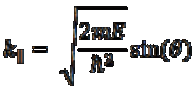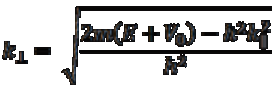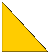Novel Materials Laboratory
 Department of Physics Department of Chemistry Division of Materials Science and Engineering
 Angle Resolved Photoemission Spectroscopy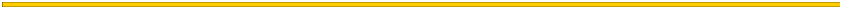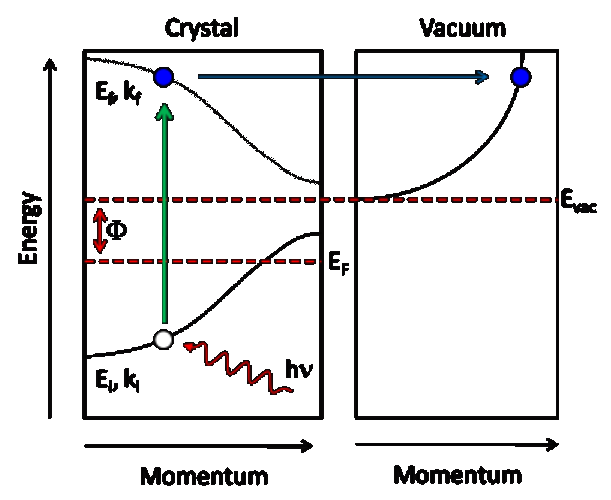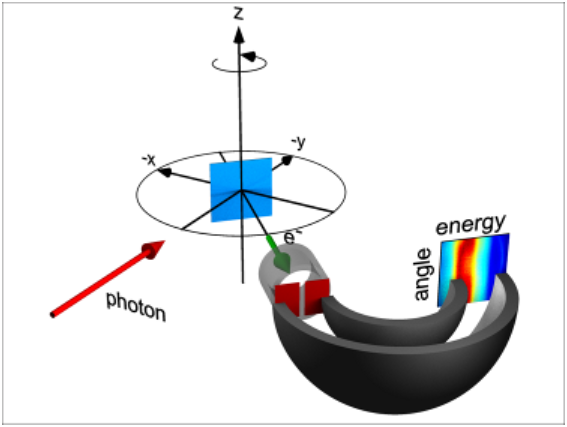Hemispherical electron energy analyzer
 Sample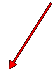EF =            Fermi energy Evac =          Vacuum level Ei , ki=        initial energy and momentum of electron in solid Ef , kf =       final energy and momentum of electron following excitation E, k =         energy and momentum of free electron in vacuum after emission F =             Evac – EF = work function hn  =           incident photon energy   Energy conservation gives E(k) = hn – F – Ei(ki) Momentum conservation parallel to the surface gives:             Momentum is not conserved perpendicular to the surface, but for a given inner potential V0, we can write:
 By measuring the kinetic energy (E) and momentum (k) of the electron in vacuum, the dispersion of the electron state E(k) in the solid can be determined